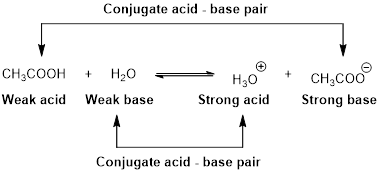Chapter 16, Problem 19PS

Chapter
Section
Textbook Problem

Which of the following ions or compounds has the strongest conjugate base? Briefly explain your choice. (a) HSO4− (b) CH3CO2H (c) HOCl

Interpretation Introduction

Interpretation:

The strongest conjugate base has to be identified from the given ions or compounds.

Concept introduction:

An equilibrium constant (K) is the ratio of concentration of products and reactants raised to appropriate stoichiometric coefficient at equlibrium.

For the general acid HA,

HA(aq)+H2O(l)H3O+(aq)+A(aq)

The relative strength of an acid and base in water can be also expressed quantitatively with an equilibrium constant as follows:

Ka=[H3O+][A][HA]

An equilibrium constant (K) with subscript a indicate that it is an equilibrium constant of an acid in water.

For the general base B,

B(aq)+H2O(l)BH(aq)+OH(aq)

The relative strength of an acid and base in water can be also expressed quantitatively with an equilibrium constant as follows:

Kb=[BH][OH][B]

An equilibrium constant (K) with subscript b indicate that it is an equilibrium constant of base in water.

Bronsted –Lowry conjugated acid-Base pairs:

When an acid is dissolved in water, the acid (HA) donates a proton to water to form a new acid (conjugated acid) and a new base (conjugated base).The pair of an Acid –Base differs by a proton called conjugated Acid-Base pair.

Explanation

Reason for the correct statement

For the general acid HA,

HA(aq)+H2O(l)H3O+(aq)+A(aq)

Ka=[H3O+][A][HA]

A large value of Ka indicates that the ionization product is strongly favoured because the product [H3O+][A] is more than the equilibrium concentration of the strong acid [HA].

A conjugate acid-base pair contains two compounds that differ only by a hydrogen ion and a charge of +1. Thus, HA and A are conjugate acid-base pair. In this pair, A is a conjugate base of an acid HA

Still sussing out bartleby?

Check out a sample textbook solution.

See a sample solution

The Solution to Your Study Problems

Bartleby provides explanations to thousands of textbook problems written by our experts, many with advanced degrees!

Get Started

T-cells are immune cells that "read" and "remember" chemical messages to identify future invaders. T F

Nutrition: Concepts and Controversies - Standalone book (MindTap Course List)

What are the chemical differences between DNA and RNA?

Biology: The Dynamic Science (MindTap Course List)

_______ is the arrival of pollen on a receptive stigma.

Biology: The Unity and Diversity of Life (MindTap Course List)